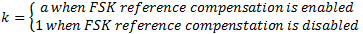# Frequency-Shift Keying (FSK) Measurements

NI-RFmx Demod 3.0 Help

Edition Date: January 2019

Part Number: 374641K-01

»View Product InfoDownload Help (Windows Only)

In frequency modulation, frequency deviation refers to the maximum difference between the instantaneous frequency of the modulated wave and the carrier frequency.

For an M-FSK system, RMS FSK error is the measure of modulation error and is defined as the RMS of the difference of the measured and the reference FSK symbols.

If fref is the transmitted symbol and freceived is the received symbol, the measured frequency symbol fmeas,n is as shown in the following equation.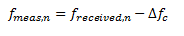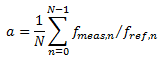where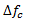is the carrier frequency error α is the gain between measured symbol and the reference symbolN is the number of symbols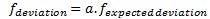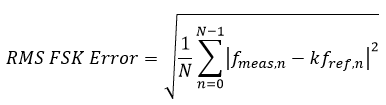where